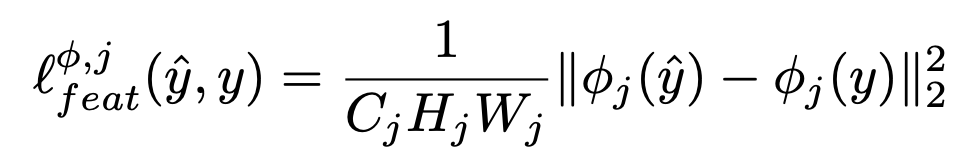# 딥러닝 논문 읽기

[Review] Perceptual Losses for Real-Time Style Transfer and Super-Resolution

2021. 6. 15. 17:41딥러닝

arxiv, github(not official)논문에선 Loss Network는 VGG-16만 씀Feature Reconstruction(Content) Loss 참고 : $\phi_{j}(\cdot)$ 는 $C_{j}\times H_{j}\times W_{j}$ dimension

Note : $\phi_{j}(\cdot)=\psi(\cdot)$ 를 $C_{j}\times D_{j}$ 로 보면, $$G_{j}^{\phi}(x)=\frac{\psi\psi^{T}}{C_{j}D_{j}}.$$

이건 uncentered covariance이다. each grid location as an independent sample(각각의 pixel=grid를 독립적으로 계산함). 그리고 captures information about which features tend to activate together(어떤 feature들이 서로 영향을 주는지에 대한 정보를 수집함).

다른 Loss(아직 공부 안함) :

VGG까지 학습함. 딱히 freeze하지는 않는것 같음.

Loss 계산 코드

# get vgg features
y_c_features = vgg(x)
y_hat_features = vgg(y_hat)

# calculate style loss
y_hat_gram = [utils.gram(fmap) for fmap in y_hat_features]
style_loss = 0.0
for j in range(4):
style_loss = STYLE_WEIGHT*style_loss
aggregate_style_loss += style_loss.data

# calculate content loss (h_relu_2_2)
recon = y_c_features
recon_hat = y_hat_features
content_loss = CONTENT_WEIGHT*loss_mse(recon_hat, recon)
aggregate_content_loss += content_loss.data

# calculate total variation regularization (anisotropic version)
# https://www.wikiwand.com/en/Total_variation_denoising
diff_i = torch.sum(torch.abs(y_hat[:, :, :, 1:] - y_hat[:, :, :, :-1]))
diff_j = torch.sum(torch.abs(y_hat[:, :, 1:, :] - y_hat[:, :, :-1, :]))
tv_loss = TV_WEIGHT*(diff_i + diff_j)
aggregate_tv_loss += tv_loss.data

# total loss
total_loss = style_loss + content_loss + tv_loss

# backprop
total_loss.backward()
optimizer.step()

#### '딥러닝' 카테고리의 다른 글

 Unsupervised Anomaly Detection vs Semi-supervised Anomaly Detection  (0) 2022.06.28 2021.06.15 2021.06.10 2021.06.10 2021.05.10 2021.04.30